# Parallelogram

• Last Updated : 10 Aug, 2022

The term Parallelogram comes from the Greek “parallelogrammon” meaning “limited by parallel lines”. So, a parallelogram is a quadrilateral surrounded by parallel lines. It has the same shape as parallel opposite sides. Parallelogram shape comes in three basic types: square, rectangular, and diamond-shaped, each with unique properties. Let’s learn the topics, what is a parallelogram, how to find the area of ​​a parallelogram, other aspects related to parallelograms, and solved examples.

## Parallelogram Definition

A parallelogram is one of the special types of quadrilateral. The angles between adjacent sides of a parallelogram can vary, but to be a parallelogram, the opposite sides must be parallel. A quadrilateral is a parallelogram if the opposite sides are parallel and equal. Therefore, a parallelogram is defined as a quadrilateral in which two pairs of opposite sides are parallel and equal.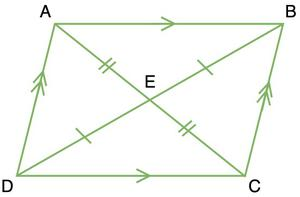## Properties of Parallelogram

There are some special properties of quadrilateral, if applied, make it a parallelogram. Let’s take a look at the properties of parallelogram,

1. The opposite sides of a parallelogram are parallel and equal.
2. The opposite angles of a parallelogram are congruent.
3. If said, one of the angles of a parallelogram is 90°. Then all the angles are 90°, and it becomes a rectangle.
4. The diagonals of the parallelogram bisect each other.
5. The consecutive angles of a parallelogram are supplementary.

## Types of Parallelogram

Parallelograms can be classified into several types based on their properties. Based on the properties of a parallelogram and on the sides and angles, the parallelogram is classified into three types,

### Rectangle

A rectangle is a parallelogram with two pairs of equal, parallel opposite sides and four right angles. Observe the rectangle ABCD and associate it with the following properties,

1. Two pairs of parallel sides. Here AB || DC and AD || BC
2. Four right angles ∠A = ∠B = ∠C = ∠D = 90°.
3. The opposite sides are the same length, where AB = DC and AD = BC.
4. Two equal diagonals where AC = BD.
5. Diagonals that bisect each other.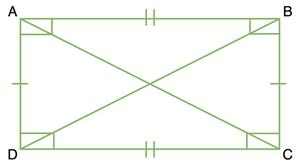### Square

A parallelogram with four equal sides and four equal angles is called a square. Observe the square ABCD and associate it with the following properties,

1. Four equal sides are AB = BC = CD = DA.
2. Right angles are ∠A = ∠B = ∠C = ∠D = 90°.
3. There are two pairs of parallel sides. Here AB || DC and AD || BC.
4. Two identical diagonals where AC = BD.
5. Diagonals are perpendicular and bisect each other; AC is perpendicular to BD.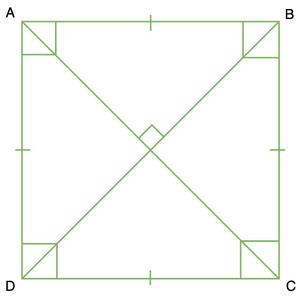### Rhombus

A parallelogram with four equal sides and equal opposite angles is called a rhombus. Consider the diamond EFGH and assign it the following attributes,

1. In the given figure, the four equal sides are EH = HG = GF = FE.
2. The two pairs of parallel sides are EH || FG and EF || HG.
3. The equal opposite angles are ∠E = ∠G and ∠H = ∠F.
4. Here, the diagonals (EG and HF) are perpendicular to each other and bisect at right angles.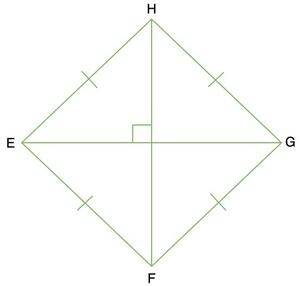All 2D shapes have two basic formulas for area and perimeter. Let’s discuss these two parallelogram formulas in this section.

## Area of Parallelogram

The area of ​​a parallelogram is the space between the four sides of the parallelogram. It can be calculated by knowing the length of the base and the height of the parallelogram and measuring it in square units such as cm2, m2, or inch2. Note the following parallelogram representing the base and height.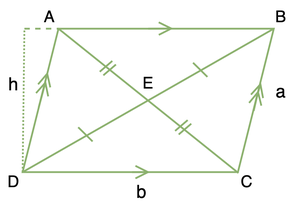Consider a parallelogram ABCD with a base (b) and a height (h). The area of ​​a parallelogram is calculated by the formula:

Parallelogram area = base (b) × height (h)

### Area of Parallelogram without Height

If the height of the parallelogram is not known, the area of the parallelogram can still be found, provided the angle is known to us. The formula for the area of parallelogram without height is given as:

Parallelogram area = ab Sinθ

Where a and b are the sides of the parallelogram and θ is the angle between them.

## Perimeter of Parallelogram

The perimeter of a parallelogram is the length of its contour, so it is equal to the sum of all sides. In a parallelogram, the opposite sides are equal. Let’s say the sides are a and b. Therefore, the perimeter (P) of a parallelogram with edges is in units of P = 2 (a + b).

Perimeter of Parallelogram = 2 (a + b)

## Parallelogram Theorem

Theorem: Parallelograms on the same base and between the same parallels have equal area.

Proof: Let’s assume two parallelograms ABCD and ABEF with the same base DC and between the same parallel lines AB and FC

To Prove: Area of parallelogram ABCD = Area of parallelogram ABEF

In the figure given below, the two parallelograms, ABCD and ABEF, lie between the same parallel lines and have the same base. Area ABDE is common between both parallelograms. Taking a closer look at the two triangles, △BCD and △AEF might be congruent.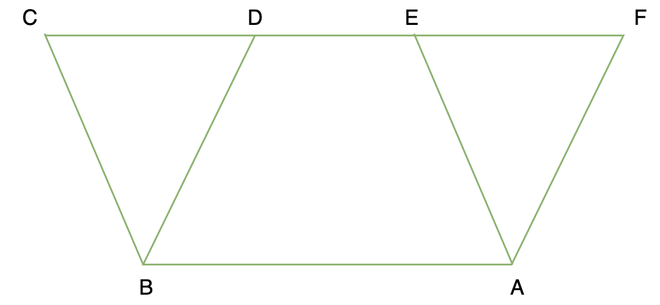BC = AE (Opposite sides of a parallelogram),

∠BCD = ∠AEF (These are corresponding angles because BC || AE and CE are the transversal).

∠BDC = ∠AFE (These are corresponding angles because BD || AF and FD are the transversals).

Thus, by the ASA criterion of congruent triangles. These two triangles are congruent, and they must have equal areas.

area(BCD) = area(AEF)

⇒ area(BCD) + area(ABDE) = area(AEF) + area(ABDE)

⇒ area(ABCD) = area(ABEF)

Hence, parallelograms lying between the same parallel lines and having a common base have equal areas.

## Solved Examples on Parallelogram

Question 1: Find the length of the other side of a parallelogram with a base of 12 cm and a perimeter of 60 cm.

Solution:

Given perimeter of a parallelogram = 60cm.

Base length of given parallelogram = 12 cm.

P = 2 (a + b) units

Where b = 12cm and P = 40cm.

60 = 2 (a + 12)

60 = 2a + 24

2a = 60-24

2a = 36

a = 18cm

Therefore, the length of the other side of the parallelogram is 18 cm.

Question 2: Find the perimeter of a parallelogram with the base and side lengths of 15cm and 5cm, respectively.

Solution:

Base length of given parallelogram = 15 cm

Side length of given parallelogram = 5 cm

Perimeter of a parallelogram is given by,

P = 2(a + b) units.

Putting the values, we get

P = 2(15 + 5)

P = 2(20)

P = 40 cm

Therefore, the perimeter of a parallelogram will be 40 cm.

Question 3: The angle between two sides of a parallelogram is 90°. If the lengths of two parallel sides are 5 cm and 4 cm, respectively, find the area.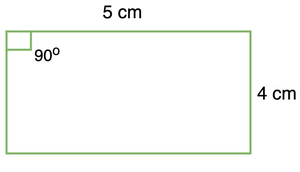Solution:

If one angle of the parallelogram is 90°. Then, the rest of the angles are also 90°. Therefore, the parallelogram becomes a rectangle. The area of the rectangle is length times breadth.

Area of parallelogram = 5 × 4

Area of parallelogram = 20cm2

Question 4: What is the area of a parallelogram when the diagonals are given as 8 cm, and 10 cm, the angle between the diagonals is 60°.

Solution:

In order to find the area of the parallelogram, the base and height should be known, lets’s first find the base of the parallelogram, applying the law of cosines,

b2 = 42 + 52 – 2(5)(4)cos(120°)

b2 = 16 + 25 – 40(0.8)

b2 = 9

b = 3cm

Finding the height of the parallelogram,4/sinθ = b/sin120

4/sinθ = 3/-0.58

sinθ = -0.773

θ = 50°

Now, to find the height,

Sinθ = h/10

0.76 = h/10

h = 7.6cm

Area of the parallelogram = 1/2 × 3 × 7.6

= 11.4 cm2

## FAQs on Parallelogram

Question 1: How can we determine the perimeter of an irregular shape?

To determine the perimeter of an irregular shape simply add the outer sides of the given shape. As perimeter is nothing but the total length of the periphery of any shape.

Question 2: How is a parallelogram different from a quadrilateral?

All parallelograms are quadrilaterals, but not all quadrilaterals are necessarily parallelograms. For example, a trapezoid is a quadrilateral, not a parallelogram. For a quadrilateral to be a parallelogram, all opposite sides must be parallel and equal.

Question 3: Is Trapezoid a parallelogram? How is a trapezoid different from a parallelogram?

A trapezoid differs from a parallelogram in that all opposite sides of the trapezoid are not parallel to each other. A trapezoid has only a pair of opposite faces that are parallel to each other.

Question 4: Do all sides of a quadrilateral have to be equal to be a parallelogram?